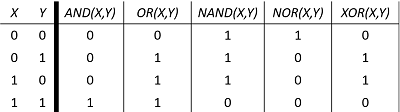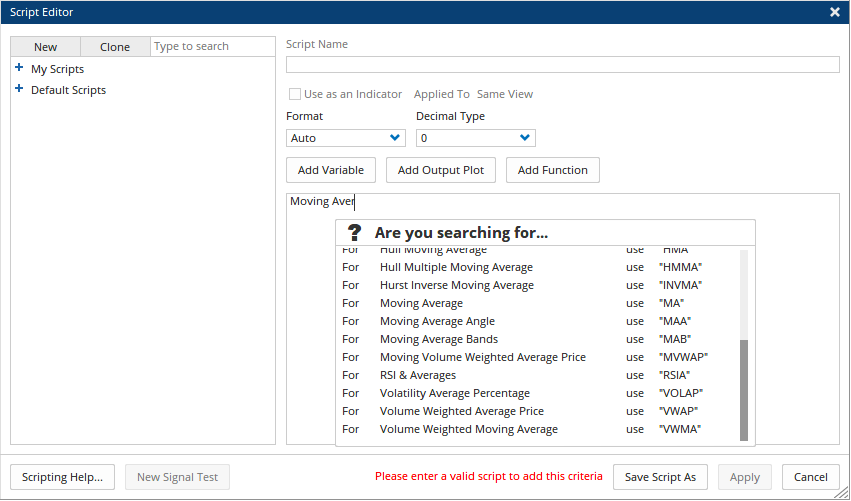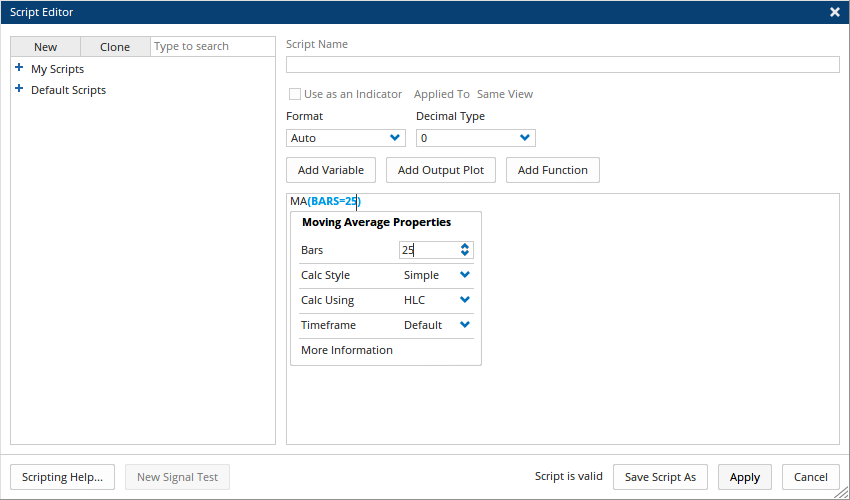# Getting Started with Optuma Scripting

Author: Optuma Team Last updated: Mar 21, 2023 14:25

## Overview

This is a quick start guide on how to write Optuma Scripts. Make sure you check out our other resources at the end of this page for more advanced examples.

Optuma Scripting can be used to build simple true / false criteria:

``````CLOSE() > MA(BARS=25)
``````

To more complex custom indicators:

``````//Set 25EMA
V1 = MA(BARS=25, STYLE=Exponential) ;
//Set Std Deviation of 25EMA
V2 = STD(V1, MULT=2.00, BARS=25);
//Plot Std Dev Upper
V3 = V1 + V2;
//Plot Std Dev Lower
V4 = V1 - V2;
//Set Rank Criteria
V5 = CLOSE() > V1 and CLOSE() < V3;
V6 = CLOSE() < V1 and CLOSE() > V4;
//Set Rank Value based on Close position between Std Lines and 25EMA using Nested IF statements<br></br>V7 = IF(V1 IsUp, IF(V5 == 1,1,2), IF(V6==1,3,4));
//Output Results
plot1 = V7;
plot1.Colour = Black;
plot1.Plotstyle = Line;
plot2 = 2;
plot2.Colour = Red;
plot2.Linestyle = Dash;
``````

There are very few limitations within Optuma Scripting, with the coding language capable of handling a large array of complex functions while keeping the interface clean and simple.

### Optuma Scripting Syntax

All coding languages have a syntax that is made up of several different components. Primarily these are broken into the following categories:

• Functions: A function, such as MA(), represents an existing calculation or indicator (in this case a Moving Average) and you can enter adjustments for the function within its parentheses. Each function has a specific adjustment syntax. For example, MA(Bars=25) would set the Moving Average function to a 25SMA.
• Constants: You can also enter constants, such as numbers, directly into a formula.
• Operators: Operators are the symbols that are used to specify the type of calculation that you want the formula to perform. For example, the * (asterisk) operator multiplies numbers.
• Variables: A variable can be set at the start of a script, to quickly reference a specific function or value. For example, V1 = MA(Bars=25) would allow you to use V1 anywhere a 25SMA needed to be referenced within the code. As scripts become longer and more complex, variables can be used to keep things simplified.
• Comments: Scripts can include different comment lines as quick references to what the code is doing at different points. To set any line within Optuma scripting as a comment, use // at the start. All comments will appear in the code editor as green text.``````//Set a Moving Average Variable for Easy Reference
V1 = MA(BARS=150) ;
//Look for instances where the close crosses above a 150SMA
Close() CrossesAbove V1
``````

### Operators Table

Standard operators such as addition (+), division (/), subtraction (-) and multiplication (*) are supported. Additionally the following operators are available within Optuma Scripting:

Operators Description
CrossesAbove Looks for instances where Function A crosses above the value of Function B (Example)
CrossesBelow Looks for instances where Function A crosses below the value of Function B (Example)
Crosses Looks for instances where Function A crosses above OR below the value of Function B (Example)
ChangeTo Can be used to signal when a value changes, eg when a variable with multiple values (eg custom rank) changes to 3: SIGNAL ChangeTo 3.

IsUp Looks for instances where Function A’s value is higher than the previous bars value.
IsDown Looks for instances where Function A’s value is lower than the previous bars value.
IsSame Looks for instances where Function A’s value is the same as the previous bars value.
TurnsUp Looks for the first instance where Function A’s value is higher than the previous bars value.
TurnsDown Looks for first instances where Function A’s value is lower than the previous bars value.
Turns Looks for first instances where Function A’s value meets the criteria for either TurnsUp or TurnsDown.
AND is a logical operator that takes two Boolean values and returns true if both values are true, and false otherwise. (Example)
OR is a logical operator that takes two Boolean values and returns true if at least one of the values is true, and false otherwise. (Example)
NAND is a logical operator that takes two Boolean values and returns false if both values are true, and true otherwise. (Example)
NOR is a logical operator that takes two Boolean values and returns true if both values are false, and false otherwise. (Example)
XOR is a logical operator that takes two Boolean values and returns true if one and only one of the values is true, and false otherwise. (Example)
== is used to compare two values and determine if they are equal or not.
> Looks for instances where Value A is greater than Value B
< Looks for instances where Value A is less than Value B
>= Looks for instances where Value A is greater than or equal to Value B
<= Looks for instances where Value A is less than or equal to Value B

Below is a quick reference guide for the logic operators available within Optuma Scripting:### Signal Scripts

Signal Scripts (also known as Booleans or True / False scripts) are coded criteria that will only produce either a True (1) or False (0) value. These types of scripts are used in many Optuma modules such as Signal Testing, Back Testing, etc. For example, a standard Back Test requires an Entry and Exit criteria, both of which must be coded as Signal Scripts.

Signal Script Example - Entry Close Crosses Above a 50EMA and Volume is Higher than the previous day

``````V1 = CLOSE() CrossesAbove MA(BARS=50, STYLE=Exponential) ;
V2 = VOLUME() IsUp ;
V1 and V2
``````

Signal Script Example - Exit
Close Crosses Below a 50EMA and Volume is Lower than the previous day

``````V1 = CLOSE() CrossesBelow MA(BARS=50, STYLE=Exponential) ;
V2 = VOLUME() IsDown ;
V1 and V2
``````

In both these examples, the output of the script is either a 1 (True) or 0 (False).

### Important Things to Note

You do not need to remember all the properties or function names to manually code within an Optuma script. Just start typing and Optuma will provide you with guidance to find what you’re after.

For example, If you are unsure of the function you wish to use, the script editor can search for the full tool name and display possible matches for you to select.In the above example, the script editor is returning the function results that match “Moving Average”.

Additionally, once a function is added to the script editor, you do not need to manually input the various properties, instead, you can click within the parentheses, to see a list of options that can be adjusted in the same manner as the tool applied to a chart.In the above example, the Moving Average function MA() is being modified to a 25SMA.

In the event the script syntax has an error, a message in red text will appear along the bottom of the editor to let you know there is an issue before the code can be saved / used.

### Optuma Scripting Resources

Scripting is a key component of many modules within Optuma, from Scanning to Alerts, Signal and Back Testing and even your own custom indicators, all use scripts to build and define what you are after.

There are many in-depth resources you will want to use if wanting to build your Optuma Scripting knowledge further.

• Optuma Scripting Video Series: This free video course is broken into 3 sections (Beginner, Intermediate, and Advanced Scripting) and should be the first stop in learning to build your own Optuma scripts. Filled with examples, and step-by-step videos you can follow along with, this series will be an invaluable resource for you. Click Here
• Optuma Scripting Functions Table: This table offers a quick list of every Optuma Scripting function you can use, with details of what they do and how they work. This is a handy page to bookmark if wanting to check whether a particular indicator is supported within Optuma Scripting. Click Here
• Optuma Scripting Forum: The most active community on the Optuma Forum is the Scripting section. Full of example scripts and advice on how to best identify various criteria or custom indicators. It is also the best place to post any questions you have if you encounter a problem getting a script to work the way you want it to. Click Here
• Optuma Scripting Knowledge Base – Script Examples: The Optuma Knowledge Base contains many script examples you can use as a base for your own custom work. If there is a script you want to build, but aren’t sure where to start, this page is a great place to check. Click Here
• Optuma Blog: The Optuma Blog regularly posts examples of different strategies and metrics built using Optuma Scripting. Click Here